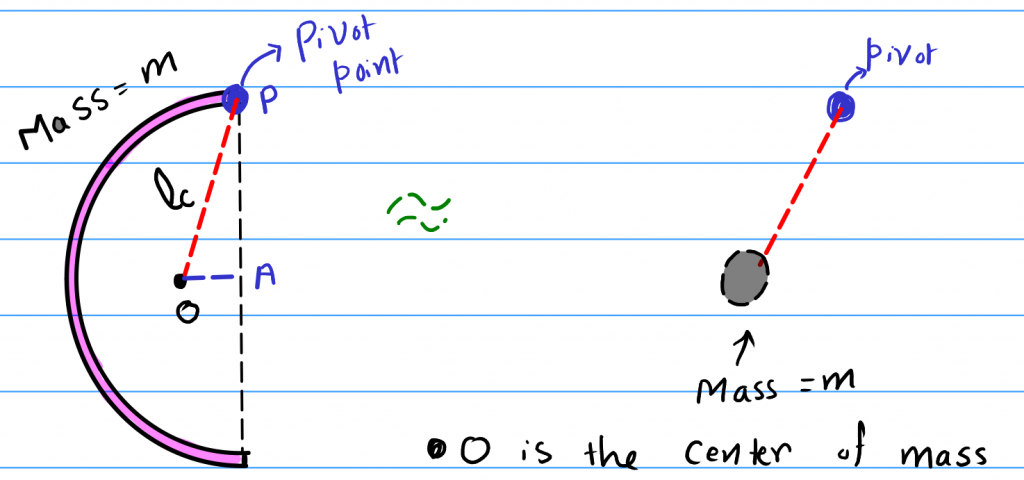How Cheenta works to ensure student success?
Explore the Back-Story

# NSEP 2015 Problem 5 | Rotational Mechanics & Small OscillationTry out this problem on the one rotational mechanics and small oscillation from National Standard Examination in Physics 2015-2016.

## NSEP 2015-16 ~ Problem 5

A thin rod of length l in the shape of a semicircle is pivoted at one of its ends such that it is free to oscillate in its own place. The frequency f of small oscillation of the semicircular rod is

(a) $\frac{1}{2\pi} \sqrt{\frac{g\pi}{2l}}$ (b) $\frac{1}{2\pi} \sqrt{\frac{g\sqrt{\pi^2+4}}{2l}}$ (c) $\frac{1}{2\pi} \sqrt{\frac{g(\pi+4)}{l}}$ (d) $\frac{1}{2\pi} \sqrt{\frac{g(\pi^2+1)}{2\pi l}}$

### Key Concepts

Rotational Mechanics and Moment of Inertia

Small Oscillations

## Suggested Book | Source | Answer

Concept of Physics H.C. Verma

University Physics by H. D. Young and R.A. Freedman

Fundamental of Physics D. Halliday, J. Walker and R. Resnick

National Standard Examination in Physics(NSEP) 2015-2016

Option-(b) $\frac{1}{2\pi} \sqrt{\frac{g\sqrt{\pi^2+4}}{2l}}$

## Try with Hints

Suppose, we have $\Gamma = -k\theta$, where $\theta$ is the angular displacement and $\Gamma$ is the torque.

Then, we can write the angular acceleration $\alpha$ as,

$$\alpha = \frac{\Gamma}{I}= -\frac{k}{I}\theta = w^2 \theta$$

where I is the moment of inertia, i.e., mass's counterpart in rotational mechanics.

Finally we have, $w = 2\pi f= \sqrt{\frac{\alpha }{\theta}} = \sqrt{\frac{\Gamma }{I\theta}}$, where f is the frequency of the oscillation.

Now for a force $\vec{F}$, $\Gamma = F r \sin(\theta)$. Now using small angle approximation $\sin(\theta) = \theta$. Hence,

$$f = \frac{1}{2\pi }\sqrt{\frac{Fr}{I}}$$

Now, we can consider the semi-circle of mass m as a point of mass m concentrated into it's centre of mass. This will give us as shown in the figure.

Now, for a semi-circle the centre of mass is at a height of $\frac{2R}{\pi}$ distance above the radius, i.e., $AO = \frac{2R}{\pi}$, where $AP=R=\frac{l}{\pi}$.

Now, $F r = mg l_{c} = mg \sqrt{R^2 + (\frac{2R}{\pi})^2}$

and $I = 2mR^2$

Putting these values gives us,

$f=\frac{1}{2 \pi} \sqrt{\frac{F r}{I}}$

=$\frac{1}{2 \pi} \sqrt{\frac{g \sqrt{\pi^{2}+4}}{2 \pi R}}$

=$\frac{1}{2 \pi} \sqrt{\frac{g \sqrt{\pi^{2}+4}}{2 l}}$

## Subscribe to Cheenta at Youtube

Try out this problem on the one rotational mechanics and small oscillation from National Standard Examination in Physics 2015-2016.

## NSEP 2015-16 ~ Problem 5

A thin rod of length l in the shape of a semicircle is pivoted at one of its ends such that it is free to oscillate in its own place. The frequency f of small oscillation of the semicircular rod is

(a) $\frac{1}{2\pi} \sqrt{\frac{g\pi}{2l}}$ (b) $\frac{1}{2\pi} \sqrt{\frac{g\sqrt{\pi^2+4}}{2l}}$ (c) $\frac{1}{2\pi} \sqrt{\frac{g(\pi+4)}{l}}$ (d) $\frac{1}{2\pi} \sqrt{\frac{g(\pi^2+1)}{2\pi l}}$

### Key Concepts

Rotational Mechanics and Moment of Inertia

Small Oscillations

## Suggested Book | Source | Answer

Concept of Physics H.C. Verma

University Physics by H. D. Young and R.A. Freedman

Fundamental of Physics D. Halliday, J. Walker and R. Resnick

National Standard Examination in Physics(NSEP) 2015-2016

Option-(b) $\frac{1}{2\pi} \sqrt{\frac{g\sqrt{\pi^2+4}}{2l}}$

## Try with Hints

Suppose, we have $\Gamma = -k\theta$, where $\theta$ is the angular displacement and $\Gamma$ is the torque.

Then, we can write the angular acceleration $\alpha$ as,

$$\alpha = \frac{\Gamma}{I}= -\frac{k}{I}\theta = w^2 \theta$$

where I is the moment of inertia, i.e., mass's counterpart in rotational mechanics.

Finally we have, $w = 2\pi f= \sqrt{\frac{\alpha }{\theta}} = \sqrt{\frac{\Gamma }{I\theta}}$, where f is the frequency of the oscillation.

Now for a force $\vec{F}$, $\Gamma = F r \sin(\theta)$. Now using small angle approximation $\sin(\theta) = \theta$. Hence,

$$f = \frac{1}{2\pi }\sqrt{\frac{Fr}{I}}$$

Now, we can consider the semi-circle of mass m as a point of mass m concentrated into it's centre of mass. This will give us as shown in the figure.

Now, for a semi-circle the centre of mass is at a height of $\frac{2R}{\pi}$ distance above the radius, i.e., $AO = \frac{2R}{\pi}$, where $AP=R=\frac{l}{\pi}$.

Now, $F r = mg l_{c} = mg \sqrt{R^2 + (\frac{2R}{\pi})^2}$

and $I = 2mR^2$

Putting these values gives us,

$f=\frac{1}{2 \pi} \sqrt{\frac{F r}{I}}$

=$\frac{1}{2 \pi} \sqrt{\frac{g \sqrt{\pi^{2}+4}}{2 \pi R}}$

=$\frac{1}{2 \pi} \sqrt{\frac{g \sqrt{\pi^{2}+4}}{2 l}}$

## Subscribe to Cheenta at Youtube

This site uses Akismet to reduce spam. Learn how your comment data is processed.

### Knowledge Partner